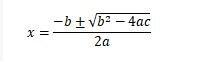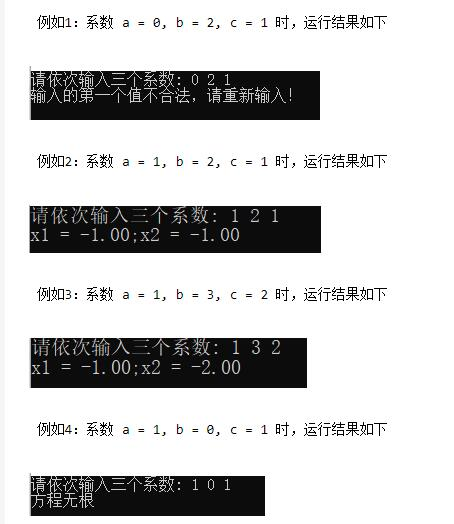2022
11-07

# c语言编程求一元二次方程的根代码思想：

#include <stdio.h>
// 使用开根号 sqrt(d) 函数时，需要添加此头文件
#include <math.h>

int main()
{
// 求一元二次方程的根
// 代码思想：
// 手动输入三个系数，分别代表二次项系数、一次项系数、常数项；
// 判断输入的二次项系数是否为0，如果为0，提示“输入的第一个值不合法，请重新输入！”
// 如果二次项系数不为0，利用根的判别式，计算一元二次方程是否有根；
// 如果判别式 Δ >= 0 ,代表方程有两个根，输出根
// 如果 Δ < 0 ,提示“方程无根”。

float a , b , c, d, x1, x2;

printf("请依次输入三个系数: ");
scanf("%f %f %f", &a,&b,&c);

if(a != 0)
{
d = b * b - 4 * a * c;                        // 根的判别式
if(d >= 0)
{
x1 = ((-b + sqrt(d)) / (2 * a));            // 求根公式
x2 = ((-b - sqrt(d)) / (2 * a));

printf("x1 = %.2f;x2 = %.2f", x1, x2);
}
else
{
printf("方程无根");
}
}
else
{
printf("输入的第一个值不合法，请重新输入！");
}

return 0;
}